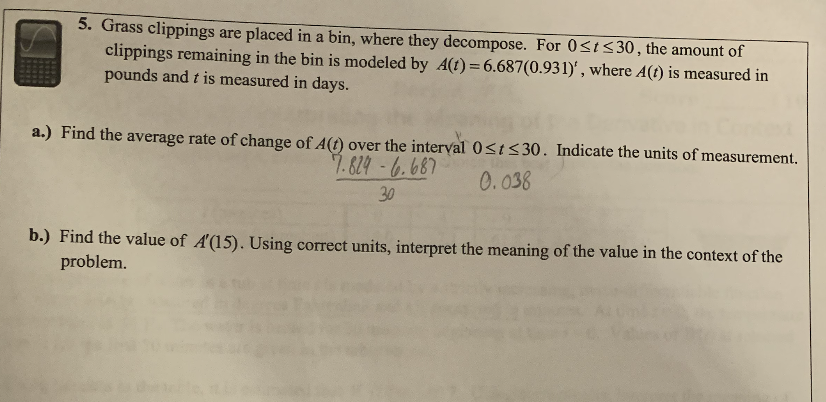Home / Expert Answers / Calculus / 5-grass-clippings-are-placed-in-a-bin-where-they-decompose-for-0-leq-t-leq-30-the-amou-pa582

# (Solved): 5. Grass clippings are placed in a bin, where they decompose. For $$0 \leq t \leq 30$$, the amou ...5. Grass clippings are placed in a bin, where they decompose. For $$0 \leq t \leq 30$$, the amount of clippings remaining in the bin is modeled by $$A(t)=6.687(0.931)^{t}$$, where $$A(t)$$ is measured in pounds and $$t$$ is measured in days. a.) Find the average rate of change of $$A(t)$$ over the interval $$0 \leq t \leq 30$$. Indicate the units of measurement. $\frac{7.824-6.687}{30} \quad 0.038$ b.) Find the value of $$A^{\prime}(15)$$. Using correct units, interpret the meaning of the value in the context of the problem.

We have an Answer from Expert